# C++虚函数与静态、动态绑定

C++继承与多态一：继承的本质和原理、访问限定表、派生类的构造过程_Rain的博客-CSDN博客

C++继承与多态二：重载，隐藏，覆盖、继承中的类型转换_Rain的博客-CSDN博客

覆盖：如果派生类中的方法，和基类继承来的某个方法，返回值、函数名、参数列表都相同，而且基类的方法是virtual虚函数，那么派生类的这个方法，自动处理成虚函数，它们之间成为覆盖关系；也就是说派生类会在自己虚函数表中将从基类继承来的虚函数进行替换，替换成派生类自己的。

静态绑定：编译时期的多态，通过函数的重载以及模板来实现，也就是说调用函数的地址在编译时期我们就可以确定，在汇编代码层次，呈现的就是 call  函数名；

动态绑定：运行时期的多态，通过派生类重写基类的虚函数来实现。在汇编代码层次，呈现的就是 call 寄存器，寄存器的值只有运行起来我们才可以确定。

# 不存在虚函数

#include <iostream>
#include <typeinfo>
class Base
{
public:
Base(int data = 10): ma(data) {}
~Base() {};

void show() {
std::cout << "Base::show()" << std::endl;
}

void show(int data) {
std::cout << "Base::show()" << data << std::endl;
}

protected:
int ma;

};

class Derive :public Base
{
public:
Derive(int data) :Base(data), mb(data) {}
~Derive() {}
void show() {
std::cout << "Derive::show()" << std::endl;
}

private:
int mb;
};

int main() {

Derive d(50);
Base *pb = &d;
pb->show();//静态（编译时期）绑定(函数调用) Base::show (06F12E4h)
pb->show(10);//Base::show (06F12BCh)

std::cout << "Base size:" << sizeof(Base) << std::endl;//4
std::cout << "Derive size:" << sizeof(Derive) << std::endl;//8

std::cout << typeid(pb).name() << std::endl;//class Base *
std::cout << typeid(*pb).name() << std::endl;//class Base

return 0;

}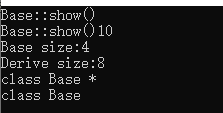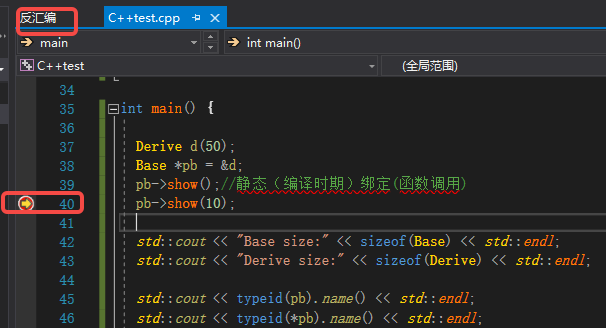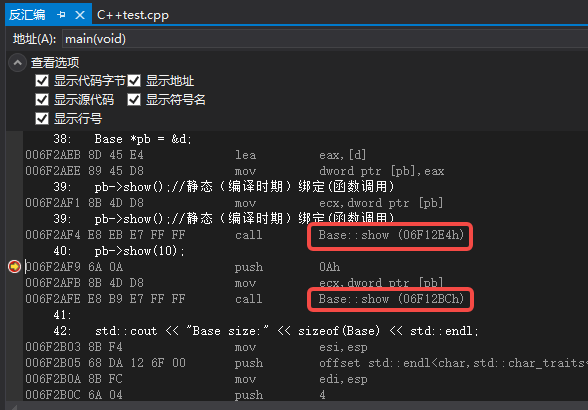可以看到调用的都是基类的show(),在编译阶段已经生成指令调用Base下的show；

pb的类型是一个class Base *；
*pb的类型是一个class Base。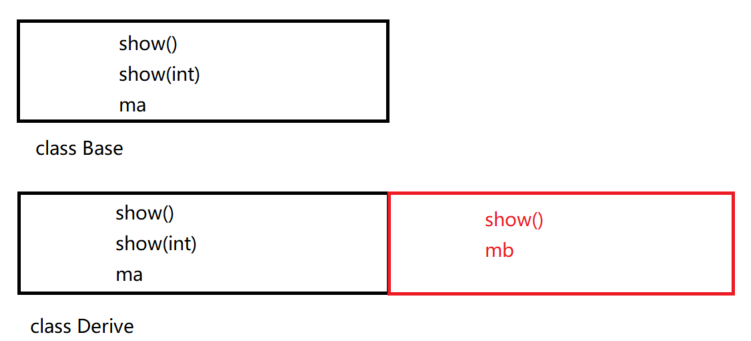Derive类继承了Base类，导致了派生类的大小要比基类大，而pb的类型是基类的指针，所以通过pb调用方法时只能访问到Derive中从Base继承而来的方法，访问不到自己重写的方法（指针的类型限制了指针解引用的能力）

# 基类定义虚函数

#include <iostream>
#include <typeinfo>
class Base
{
public:
Base(int data = 10): ma(data) {}
~Base() {};

//虚函数
virtual void show() {
std::cout << "Base::show()" << std::endl;
}

void show(int data) {
std::cout << "Base::show()" << data << std::endl;
}

protected:
int ma;

};

class Derive :public Base
{
public:
Derive(int data) :Base(data), mb(data) {}
~Derive() {}
void show() {
std::cout << "Derive::show()" << std::endl;
}

private:
int mb;
};

int main() {

Derive d(50);
Base *pb = &d;

/*

pb->show();
pb 指针是base类型，如果发现Base中的show是虚函数，就进行动态绑定
mov         ecx,dword ptr [pb]
00292B01 8B 45 D4             mov         eax,dword ptr [pb]   //将pb指向的内存前4个字节放入ecx寄存器，pb指向derive对象，前四个字节即vfptr，将虚函数表地址加载到eax
00292B04 8B 10                mov         edx,dword ptr [eax]  //将eax 的前四个字节 即Derive::show 加载到edx中
00292B06 8B F4                mov         esi,esp
00292B08 8B 4D D4             mov         ecx,dword ptr [pb]
00292B0B 8B 02                mov         eax,dword ptr [edx]
00292B0D FF D0                call        eax   //虚函数的地址
00292B0F 3B F4                cmp         esi,esp
00292B11 E8 9C E7 FF FF       call        __RTC_CheckEsp (02912B2h)

eax寄存器里存放的是什么内容，编译阶段根本无从知晓，只能在运行的时候确定；

pb->show(10);  如果发现show是普通函数，就进行静态绑定 call Base::show

*/
pb->show();//
pb->show(10);//

std::cout << "Base size:" << sizeof(Base) << std::endl;//8
std::cout << "Derive size:" << sizeof(Derive) << std::endl;//12

std::cout << typeid(pb).name() << std::endl;//class Base *
/*
pb的类型：Base类型，查看Base中有没有虚函数
（1）Base中没有虚函数*pb识别的就是编译时期的类型 *pb 就是Base类型
(2) Base中有虚函数，*pb识别的就是运行时期的类型 RTTI类型:Derive
*/
std::cout << typeid(*pb).name() << std::endl;//class Derive

return 0;

}

• 首先，如果类里面定义了虚函数，那么编译阶段，编译器给这个类类型产生一个唯一的vftable虚函数表，虚函数表中主要存储的内容是：RTTI(Run-time Type Information)指针和虚函数的地址，当程序运行时，每一张虚函数表都会加载到内存的.rodata区；
• 一个类里面定义了虚函数，那么这个类定义的对象，在运行时，内存中会多存储一个vfptr虚函数指针，指向了对应类型的虚函数表vftable；
• 一个类型定义的n个对象，他们的vfptr指向的都是同一张虚函数表；
• 一个类里面虚函数的个数，不影响对象内存的大小(vfptr)，影响的是虚函数表的大小。
• 如果派生类中的方法和从基类继承来的某个方法中返回值、函数名以及参数列表都相同，且基类的方法是virtual，那么派生类的这个方法，自动处理成虚函数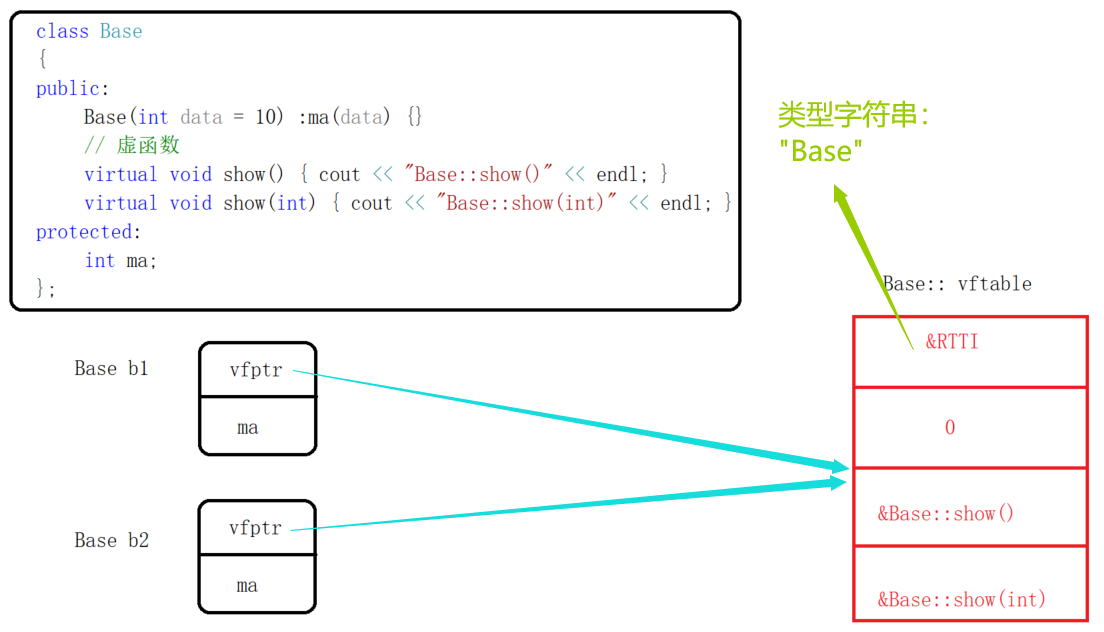1、RTTI，存放的是类型信息，也就是（Base或者Derive）
2、偏移地址：虚函数指针相对于对象内存空间的偏移，一般vfptr都在0偏移位置
3、下面的函数时虚函数入口地址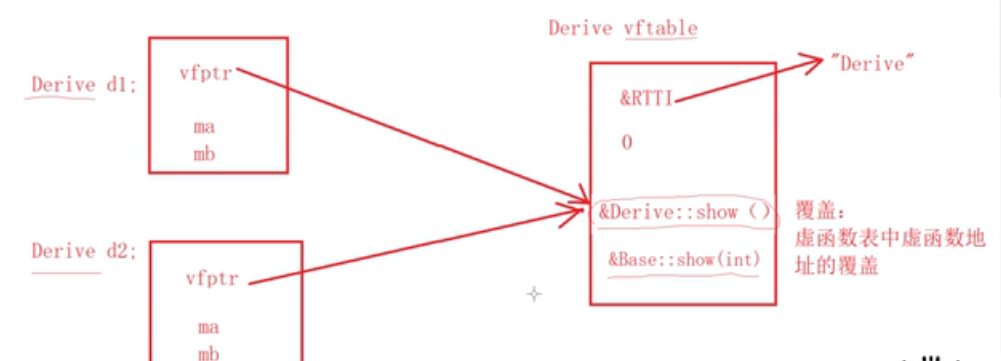在Derive类中，由于重写了show(),因此在Derive的虚函数表中，是使用子类的show()方法代替了Base类的show()

### VS的工具来查看虚函数表的有关信息

1 找到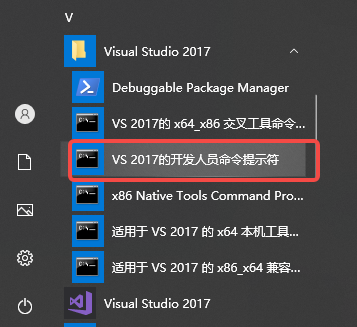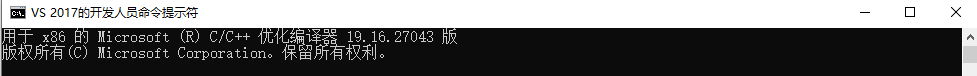2 在打开的窗口中切换到当前工程所在目录：

C:\Program Files (x86)\Microsoft Visual Studio\2017\Community>cd  C:\Users\Admin\source\repos\C++test\

3 输入命令：cl XXX.cpp /d1reportSingleClassLayoutXX(第一个XXX表示源文件的名字，第二个代表你想查看的类类型，我这里就是Derive)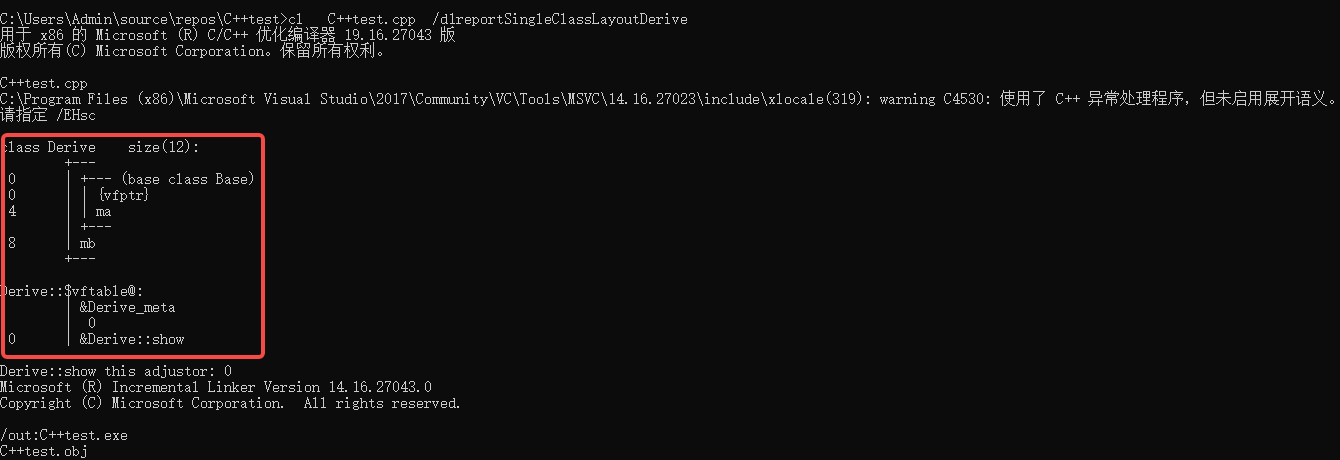# 多重继承、多继承 的虚函数表

## 1 内存分布

(1) ClassA *a = new ClassB();
(2) ClassB b; ClassA *a = &b;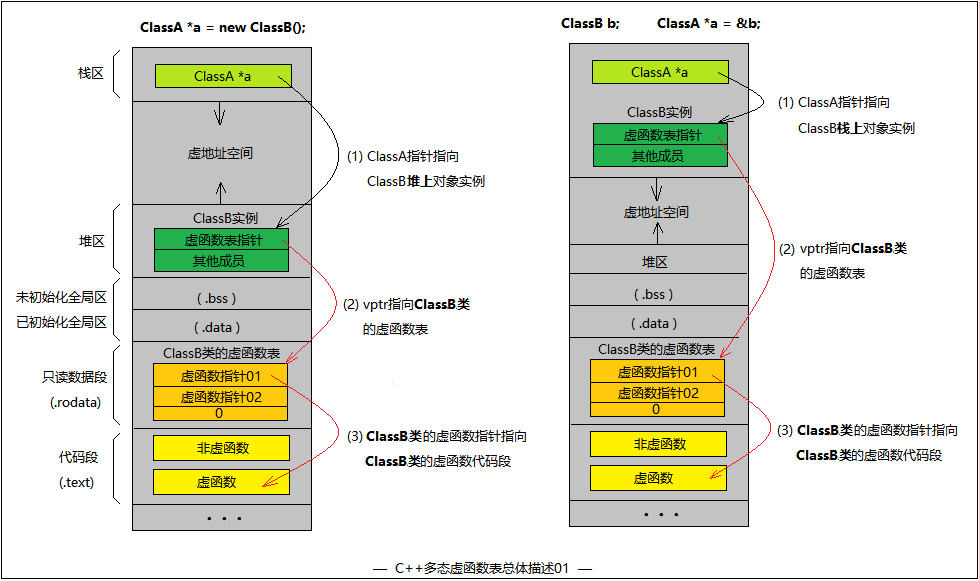## 2 类的虚函数表与类实例的虚函数指针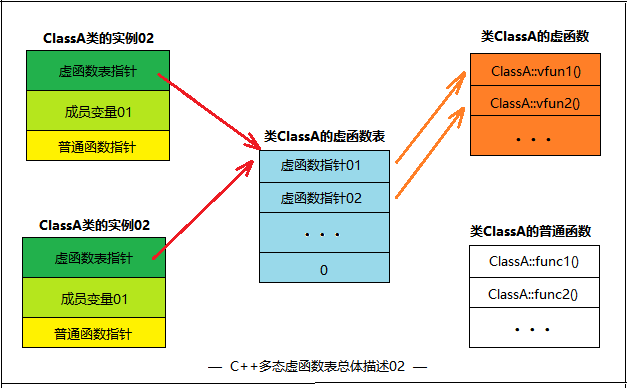## 3 多态代码及多重继承情况

#include <iostream>

using namespace std;

class ClassA
{
public:
ClassA() { cout << "ClassA::ClassA()" << endl; }
virtual ~ClassA() { cout << "ClassA::~ClassA()" << endl; }

void func1() { cout << "ClassA::func1()" << endl; }
void func2() { cout << "ClassA::func2()" << endl; }

virtual void vfunc1() { cout << "ClassA::vfunc1()" << endl; }
virtual void vfunc2() { cout << "ClassA::vfunc2()" << endl; }
private:
};

class ClassB : public ClassA
{
public:
ClassB() { cout << "ClassB::ClassB()" << endl; }
virtual ~ClassB() { cout << "ClassB::~ClassB()" << endl; }

void func1() { cout << "ClassB::func1()" << endl; }
virtual void vfunc1() { cout << "ClassB::vfunc1()" << endl; }
private:
int bData;
};

class ClassC : public ClassB
{
public:
ClassC() { cout << "ClassC::ClassC()" << endl; }
virtual ~ClassC() { cout << "ClassC::~ClassC()" << endl; }

void func2() { cout << "ClassC::func2()" << endl; }
virtual void vfunc2() { cout << "ClassC::vfunc2()" << endl; }
private:
int cData;
};

int main()
{
ClassC c;

return 0;
}


(1) ClassA是基类, 有普通函数: func1() func2() 。虚函数: vfunc1() vfunc2() ~ClassA()
(2) ClassB继承ClassA, 有普通函数: func1()。虚函数: vfunc1() ~ClassB()
(3) ClassC继承ClassB, 有普通函数: func2()。虚函数: vfunc2() ~ClassB()

ClassB继承与ClassA，其虚函数表是在ClassA虚函数表的基础上有所改动的，变化的仅仅是在子类中重写的虚函数。如果子类没有重写任何父类虚函数，那么子类的虚函数表和父类的虚函数表在内容上是一致的

ClassA *a = new ClassB();
a->func1();                    // "ClassA::func1()"   隐藏了ClassB的func1()
a->func2();                    // "ClassA::func2()"
a->vfunc1();                   // "ClassB::vfunc1()"  重写了ClassA的vfunc1()
a->vfunc2();                   // "ClassA::vfunc2()"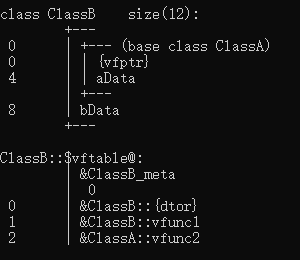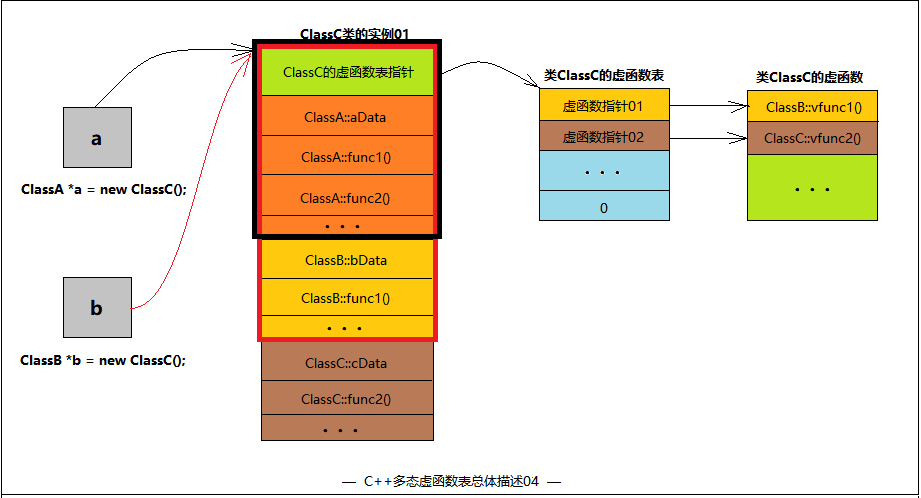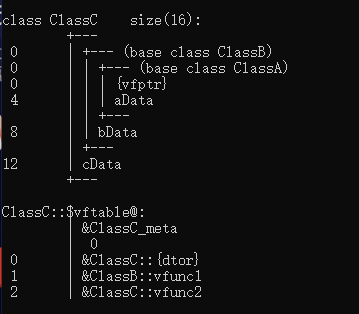## 4、多继承下的虚函数表 (同时继承多个基类)

#include <iostream>

using namespace std;

class ClassA1
{
public:
ClassA1() { cout << "ClassA1::ClassA1()" << endl; }
virtual ~ClassA1() { cout << "ClassA1::~ClassA1()" << endl; }

void func1() { cout << "ClassA1::func1()" << endl; }

virtual void vfunc1() { cout << "ClassA1::vfunc1()" << endl; }
virtual void vfunc2() { cout << "ClassA1::vfunc2()" << endl; }
private:
int a1Data;
};

class ClassA2
{
public:
ClassA2() { cout << "ClassA2::ClassA2()" << endl; }
virtual ~ClassA2() { cout << "ClassA2::~ClassA2()" << endl; }

void func1() { cout << "ClassA2::func1()" << endl; }

virtual void vfunc1() { cout << "ClassA2::vfunc1()" << endl; }
virtual void vfunc2() { cout << "ClassA2::vfunc2()" << endl; }
virtual void vfunc4() { cout << "ClassA2::vfunc4()" << endl; }
private:
int a2Data;
};

class ClassC : public ClassA1, public ClassA2
{
public:
ClassC() { cout << "ClassC::ClassC()" << endl; }
virtual ~ClassC() { cout << "ClassC::~ClassC()" << endl; }

void func1() { cout << "ClassC::func1()" << endl; }

virtual void vfunc1() { cout << "ClassC::vfunc1()" << endl; }
virtual void vfunc2() { cout << "ClassC::vfunc2()" << endl; }
virtual void vfunc3() { cout << "ClassC::vfunc3()" << endl; }
};

int main()
{
ClassC c;

return 0;
}


ClassA1是第一个基类，拥有普通函数func1()，虚函数vfunc1() vfunc2()。
ClassA2是第二个基类，拥有普通函数func1()，虚函数vfunc1() vfunc2()，vfunc4()。
ClassC依次继承ClassA1、ClassA2。普通函数func1(),虚函数vfunc1() vfunc2() vfunc3()。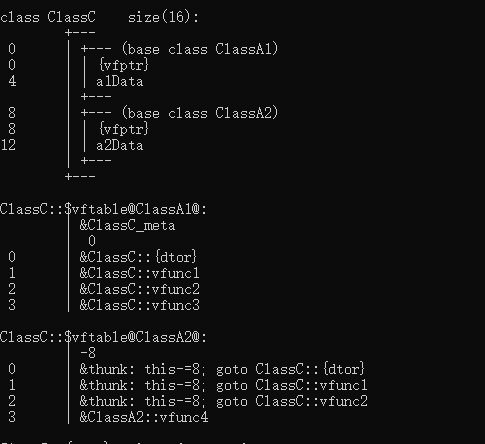在多继承情况下，有多少个基类就有多少个虚函数表指针，前提是基类要有虚函数才算上这个基类。

1.子类虚函数会覆盖每一个父类的每一个同名虚函数。
2.父类中没有的虚函数而子类有，填入第一个虚函数表中，且用父类指针是不能调用。
3.父类中有的虚函数而子类没有，则不覆盖。仅子类和该父类指针能调用

# 虚基类和多重继承

## 什么是多重继承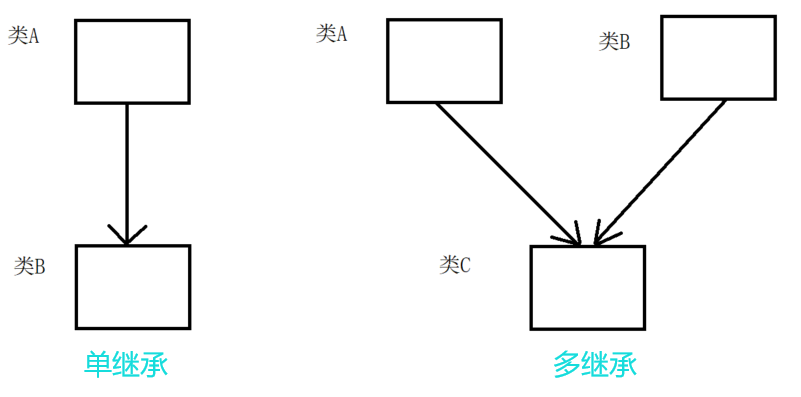类B类C类A单继承而来；
类D类B类C多继承而来。

#include <iostream>

using namespace std;
class A
{
public:
A(int data) :ma(data) { cout << "A()" << endl; }
~A() { cout << "~A()" << endl; }
protected:
int ma;
};
class B :public A
{
public:
B(int data) :A(data), mb(data) { cout << "B()" << endl; }
~B() { cout << "~B()" << endl; }
protected:
int mb;
};
class C :public A
{
public:
C(int data) :A(data), mc(data) { cout << "C()" << endl; }
~C() { cout << "~C()" << endl; }
protected:
int mc;
};
class D :public B, public C
{
public:
D(int data) : B(data), C(data), md(data) { cout << "D()" << endl; }
~D() { cout << "~D()" << endl; }
protected:
int md;
};
int main()
{
D d(10);

return 0;
}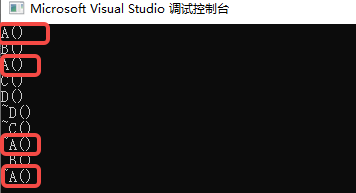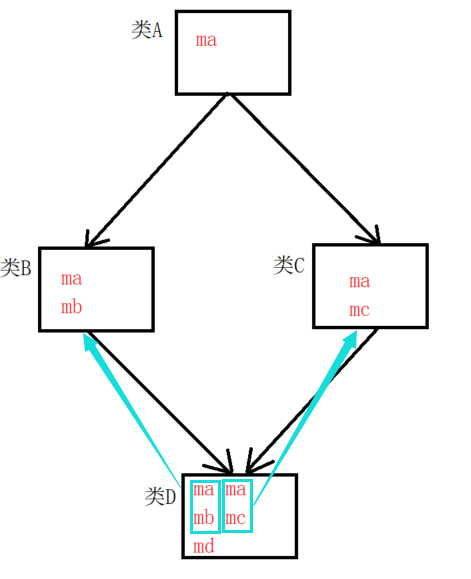（如果多继承的数量增加，那么派生类中重复的数据也会增加！）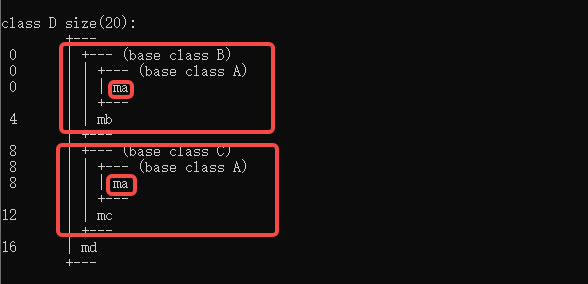### 其他多重继承的情况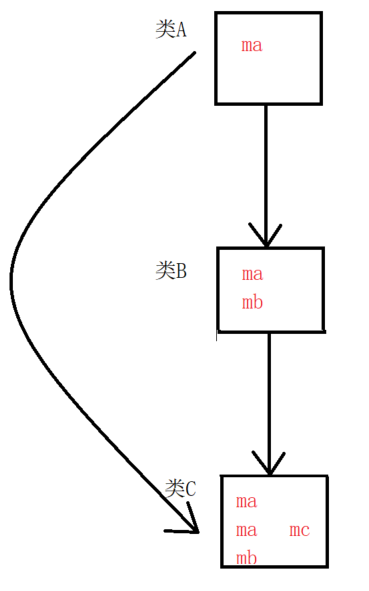## 如何解决多重继承的问题

class A
{
XXXXXX;
};
class B : virtual public A
{
XXXXXX;
};


对于这个示例而言，B虚继承了A，所以把A称作虚基类。

### 虚基类如何解决问题

#include <iostream>

using namespace std;
class A
{
public:
A(int data) :ma(data) { cout << "A()" << endl; }
~A() { cout << "~A()" << endl; }
protected:
int ma;
};
class B :virtual public A
{
public:
B(int data) :A(data), mb(data) { cout << "B()" << endl; }
~B() { cout << "~B()" << endl; }
protected:
int mb;
};
class C :virtual public A
{
public:
C(int data) :A(data), mc(data) { cout << "C()" << endl; }
~C() { cout << "~C()" << endl; }
protected:
int mc;
};
class D :public B, public C
{
public:
D(int data) : B(data), C(data), md(data) { cout << "D()" << endl; }
~D() { cout << "~D()" << endl; }
protected:
int md;
};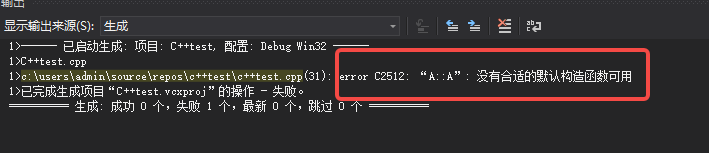提示说："A::A" : 没有合适的默认构造函数可用

D(int data) :A(data), B(data), C(data), md(data) { cout << "D()" << endl; }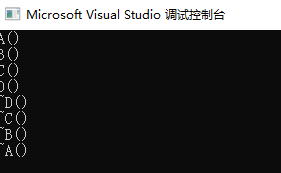### 查看虚基类的内存布局

我们可以看到当前B的内存空间：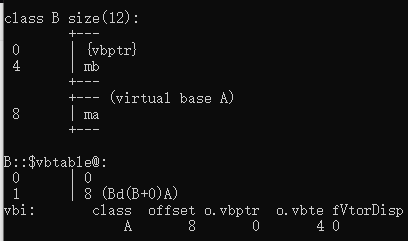vfptr（虚函数指针）指向了vftable（虚函数表）一样，
vbptr（虚基类指针）指向了vbtable（虚基类表）。

vbtable（虚基类表）的布局也如图所示，

### 对比普通继承下的内存布局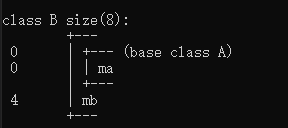我们把他们放在一起对比可以看到：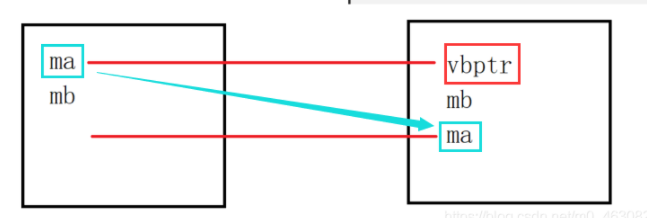继承虚基类的类（BC）会把自己从虚基类继承而来的数据ma放在自己内存的最末尾（偏移量最大），并在原来ma的位置填充一个vbptr（虚基类指针），这个指针指向了vbtable（虚基类表）。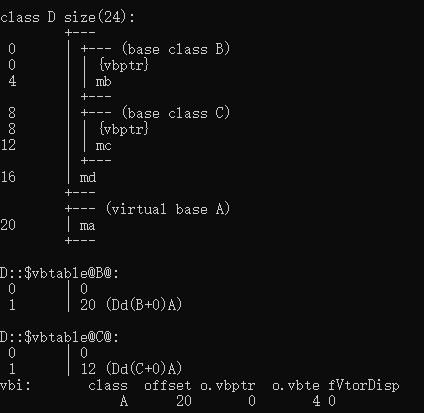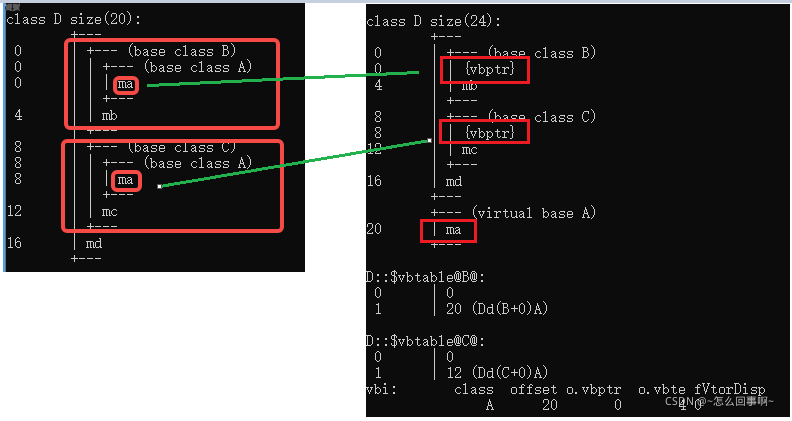可以看到，将ma移动到了末尾处，并在含有ma的地方，都用vbptr进行填充。

# 虚析构函数

基类的指针(引用)指向堆上new出来的派生类对象的时候，delete调用析构函数的时候，必须发生动态绑定，否则会导致派生类的析构函数无法调用。

#include <iostream>
using namespace std;

class Base
{
public:
Base(int data) :ma(data)
{
cout << "Base()" << endl;
}
~Base()
{
cout << "~Base()" << endl;
}
virtual void show()
{
cout << "call Base::show()" << endl;
}
protected:
int ma;
};

class Derive : public Base
{
public:
Derive(int data) :Base(data), mb(data), ptr(new int(data))
{
cout << "Derive()" << endl;
}
~Derive()
{
delete ptr;
cout << "~Derive() " << endl;
}
private:
int mb;
int *ptr;
};

int main()
{
Base *pb = new Derive(10);
pb->show();//静态绑定pb Base*   *pb Derive
delete pb;

return 0;
}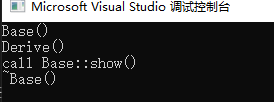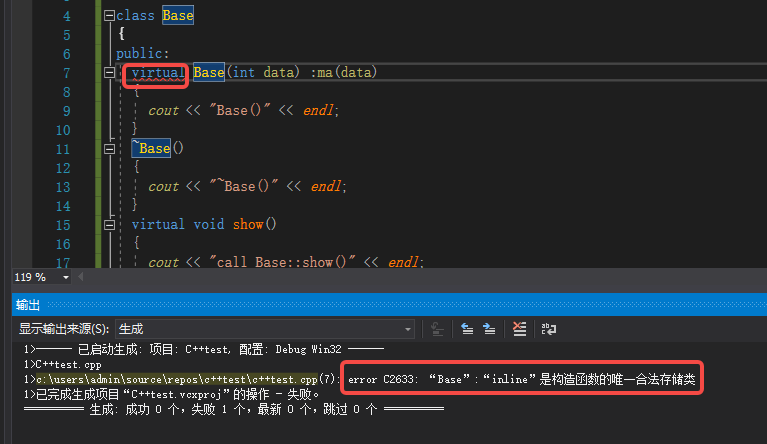（1）从存储空间角度

虚函数对应一个vtable，这大家都知道，可是这个vtable其实是存储在对象的内存空间的。问题出来了，如果构造函数是虚的，就需要通过 vtable来调用，可是对象还没有实例化，也就是内存空间还没有，怎么找vtable呢？所以构造函数不能是虚函数。

（2）从使用角度

（3）虚函数的作用在于通过父类的指针或者引用来调用它的时候能够变成调用子类的那个成员函数。而构造函数是在创建对象时自动调用的，不可能通过父类的指针或者引用去调用，因此也就规定构造函数不能是虚函数。

（4）构造函数不需要是虚函数，也不允许是虚函数，因为创建一个对象时我们总是要明确指定对象的类型，尽管我们可能通过实验室的基类的指针或引用去访问它。但析构却不一定，我们往往通过基类的指针来销毁对象。这时候如果析构函数不是虚函数，就不能正确识别对象类型从而不能正确调用析构函数。

（5）从实现上看，vbtl在构造函数调用后才建立，因而构造函数不可能成为虚函数

# 深入动态绑定问题

1.用对象本身调用虚函数，是静态绑定
2.动态绑定：虚函数前面必须是指针或引用调用才能发生动态绑定：基类指针指向基类对象，基类指针指向派生类对象，都是动态绑定。
3.如果不是通过指针或者引用来调用虚函数，那就是静态绑定

### 案例1：静态绑定

class Base
{
public:
Base(int data = 0):ma(data){}
virtual void show()
{
cout << "Base::show()" << endl;
}
protected:
int ma;
};

class Derive : public Base
{
public:
Derive(int data = 0):Base(data), mb(data){}
void show()
{
cout << "Derive::show()" << endl;
}
private:
int mb;
};

int main()
{
Base b;
Derive d;

//静态绑定
b.show();//虚函数 call Base::show();
d.show();//虚函数 call Derive::show();

return 0;
}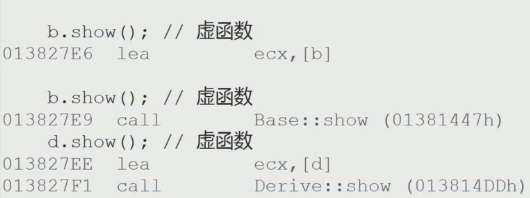### 案例2：基类指针指向基类对象

Base b;
Derive d;

Base *pb1 = &b;//基类指针指向基类对象
pb1->show();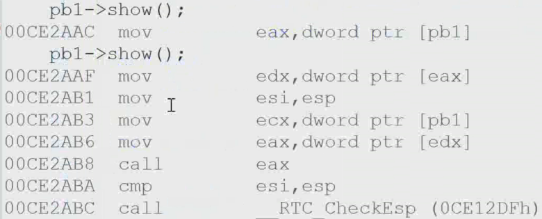### 案例3：基类指针指向派生类对象

Base b;
Derive d;

Base *pb2 = &d;//基类指针指向派生类对象
pb2->show();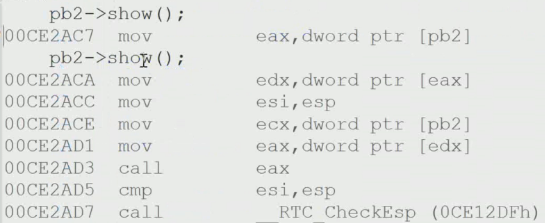转到反汇编：为动态绑定

### 案例4：基类指针指向基类对象，基类指针引用派生类对象

Derive *pd1 = &d;
pd1->show();
Derive &rd1 = d;
rd1.show();


### 案例5：派生类指针调用派生类对象，派生类引用调用派生类对象

Derive *pd1 = &d;
pd1->show();
Derive &rd1 = d;
rd1.show();


### 案例6：强制类型转换

Derive *pd2 = (Derive*)&b;
pd2->show();


07-17441
09-08634
09-021103
06-071293
11-01486
08-14180
11-07315
04-191471
05-06598
12-222132
06-06880
05-15108
12-181124
02-23195
05-177万+
05-225015
12-124725

### “相关推荐”对你有帮助么？

•非常没帮助
•没帮助
•一般
•有帮助
•非常有帮助被折叠的  条评论 为什么被折叠?到【灌水乐园】发言~怎么回事啊~

¥2 ¥4 ¥6 ¥10 ¥20余额支付 (余额：-- )扫码支付获取中扫码支付点击重新获取扫码支付1.余额是钱包充值的虚拟货币，按照1:1的比例进行支付金额的抵扣。
2.余额无法直接购买下载，可以购买VIP、C币套餐、付费专栏及课程。余额充值